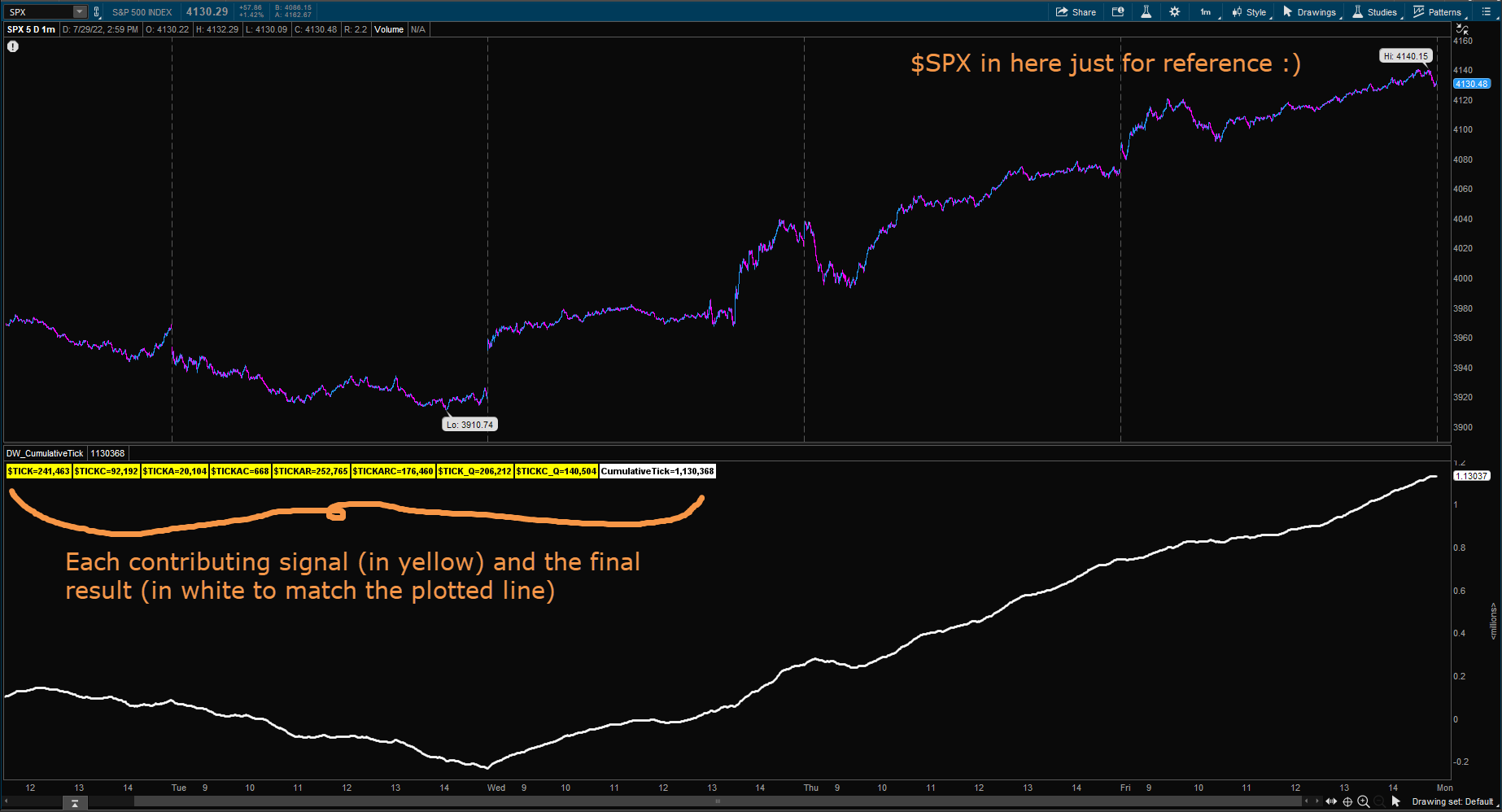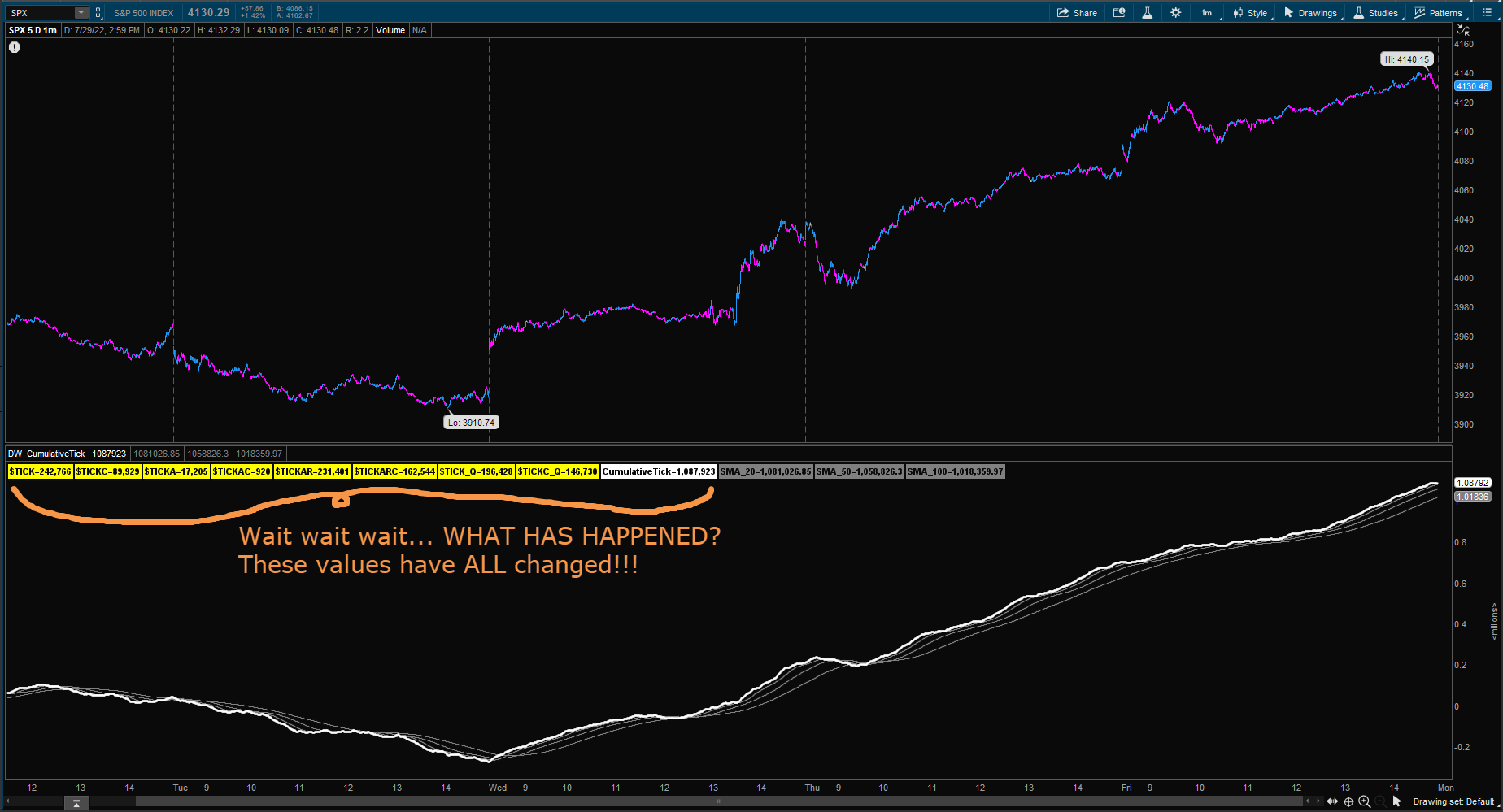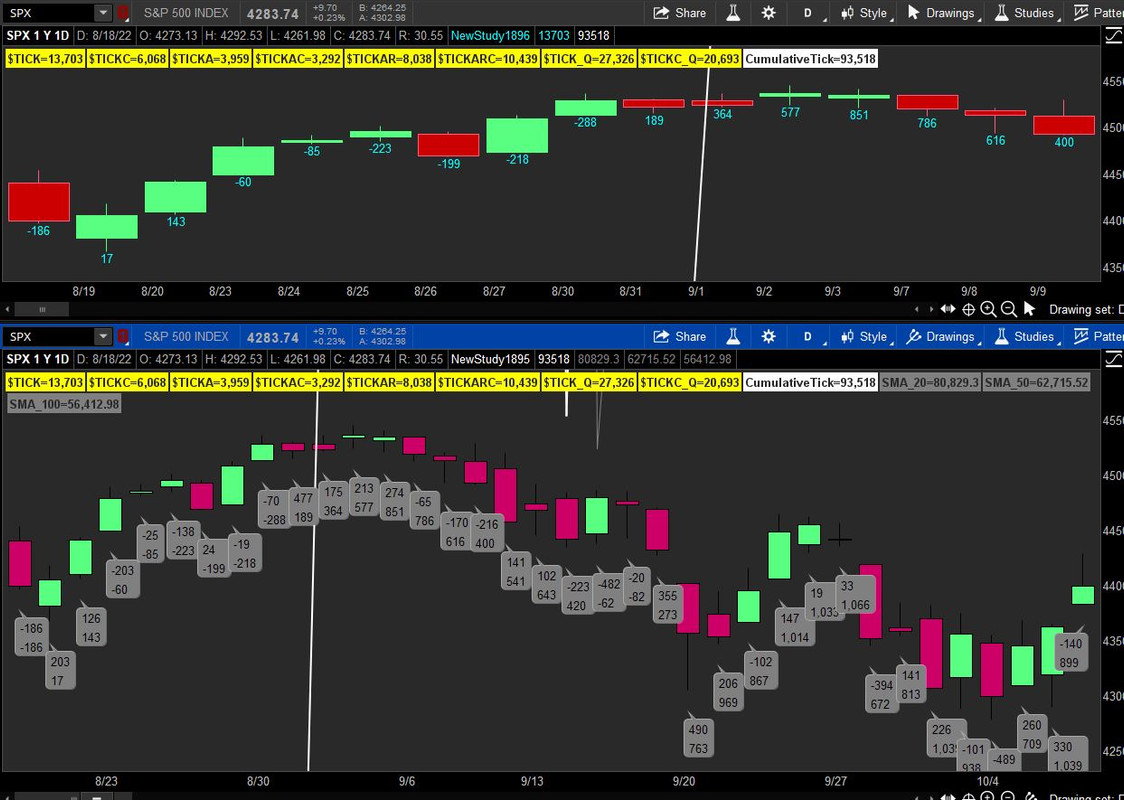# Cumulative Tick "underlying" value changes when adding moving averages?

#### danwagnerco

##### New member
VIP
I was inspired by these two posts to create a "Cumulative Tick" signal:

Except, instead of resetting the "Cumulative Tick" value each day, I would instead let it constantly accumulate to assess long-term trends. Using the TOS-provided documentation on these symbols as my guide:

https://tlc.thinkorswim.com/center/release/rel-07-20-2013

I decided to sum ALL of \$TICK-style sources to take in a truly global view. Here is the super-basic code to accomplish that, with Labels added to easily assess the values of each signal:

Code:
``````# BenTen \$TICK accumulator: https://usethinkscript.com/threads/cumulative-tick-indicator-for-thinkorswim.603/

def TICK = close(Symbol = "\$TICK");
def cumTICK = cumTICK + TICK;
def TICKC = close(Symbol = "\$TICKC");
def cumTICKC = cumTICKC + TICKC;
def TICKA = close(Symbol = "\$TICKA");
def cumTICKA = cumTICKA + TICKA;
def TICKAC = close(Symbol = "\$TICKAC");
def cumTICKAC = cumTICKAC + TICKAC;
def TICKAR = close(Symbol = "\$TICKAR");
def cumTICKAR = cumTICKAR + TICKAR;
def TICKARC = close(Symbol = "\$TICKARC");
def cumTICKARC = cumTICKARC + TICKARC;
def TICK_Q = close(Symbol = "\$TICK/Q");
def cumTICK_Q = cumTICK_Q + TICK_Q;
def TICKC_Q = close(Symbol = "\$TICKC/Q");
def cumTICKC_Q = cumTICKC_Q + TICKC_Q;

def totalCumTick = cumTICK + cumTICKC + cumTICKA + cumTICKAC + cumTICKAR + cumTICKARC + cumTICK_Q + cumTICKC_Q;

plot tct = totalCumTick;
tct.SetLineWeight(2);
tct.SetPaintingStrategy(PaintingStrategy.LINE);
tct.AssignValueColor(Color.WHITE);``````

Here is the result:OK, now that I have my signal, let's slap on some moving averages -- perhaps I can conclude that the market is short-term bullish or bearish when this "Cumulative Tick" value is above its 20-bar moving average / 50-bar moving average / 100-bar moving average etc. I will add those calculations and also add them as labels to the calculated values are easy to see:

Code:
``````# ... exact same code from above...
# Here is the new stuff:
def SMA_1 = MovingAverage(AverageType.Simple, totalCumTick, 20);
def SMA_2 = MovingAverage(AverageType.Simple, totalCumTick, 50);
def SMA_3 = MovingAverage(AverageType.Simple, totalCumTick, 100);

plot sma1 = SMA_1;
sma1.SetLineWeight(1);
sma1.SetPaintingStrategy(PaintingStrategy.LINE);
sma1.AssignValueColor(Color.GRAY);
plot sma2 = SMA_2;
sma2.SetLineWeight(1);
sma2.SetPaintingStrategy(PaintingStrategy.LINE);
sma2.AssignValueColor(Color.GRAY);
plot sma3 = SMA_3;
sma3.SetLineWeight(1);
sma3.SetPaintingStrategy(PaintingStrategy.LINE);
sma3.AssignValueColor(Color.GRAY);``````

I save the updated Thinkscript and here are the results:ALL of the "underlying" signals, the raw \$TICKXYZ values, have changed after adding these simple moving averages! This runs counter to years of programming experience -- I am writing this on Sunday Jul22 31st, at 10:45AM Chicago time, so all markets are closed and these values should not be changing.

Why has the addition of simple moving averages beyond the underlying signals changed the underlying signals?

Thank you so much in advance for your help here my friends as I feel like I'm taking crazy pills haha... -Dan

PS, for reference, the values before and after the addition of the simple moving averages:

Before:
\$TICK=241_463
\$TICKC=92_192
\$TICKA=20_104
\$TICKAC=668
\$TICKAR=252_765
\$TICKARC=176_460
\$TICK/Q=206_212
\$TICKC/Q=140_504
CumulativeTick=1_130_368

After:
\$TICK=242_766 (+0.54% larger)
\$TICKC=89_929 (-2.45% smaller)
\$TICKA=17_205 (-14.42% smaller)
\$TICKAC=920 (+37.72% larger)
\$TICKAR=231_401 (-8.45% smaller)
\$TICKARC=162_544 (-7.89% smaller)
\$TICK/Q=196_428 (-4.74% smaller)
\$TICKC/Q=146_730 (+4.43% larger)
CumulativeTick=1_087_923 (-3.75% smaller)

Solution
I was inspired by these two posts to create a "Cumulative Tick" signal:

Except, instead of resetting the "Cumulative Tick" value each day, I would instead let it constantly accumulate to assess long-term trends. Using the TOS-provided documentation on these symbols as my guide:

https://tlc.thinkorswim.com/center/release/rel-07-20-2013

I decided to sum ALL of \$TICK-style sources to take in a truly global view. Here is the super-basic code to accomplish that, with Labels added to easily assess the values of...
I tried to make this code work, but I get no values showing. Can you post the complete code for this please. Thank you.

I was inspired by these two posts to create a "Cumulative Tick" signal:

Except, instead of resetting the "Cumulative Tick" value each day, I would instead let it constantly accumulate to assess long-term trends. Using the TOS-provided documentation on these symbols as my guide:

https://tlc.thinkorswim.com/center/release/rel-07-20-2013

I decided to sum ALL of \$TICK-style sources to take in a truly global view. Here is the super-basic code to accomplish that, with Labels added to easily assess the values of each signal:

Code:
``````# BenTen \$TICK accumulator: https://usethinkscript.com/threads/cumulative-tick-indicator-for-thinkorswim.603/

def TICK = close(Symbol = "\$TICK");
def cumTICK = cumTICK + TICK;
def TICKC = close(Symbol = "\$TICKC");
def cumTICKC = cumTICKC + TICKC;
def TICKA = close(Symbol = "\$TICKA");
def cumTICKA = cumTICKA + TICKA;
def TICKAC = close(Symbol = "\$TICKAC");
def cumTICKAC = cumTICKAC + TICKAC;
def TICKAR = close(Symbol = "\$TICKAR");
def cumTICKAR = cumTICKAR + TICKAR;
def TICKARC = close(Symbol = "\$TICKARC");
def cumTICKARC = cumTICKARC + TICKARC;
def TICK_Q = close(Symbol = "\$TICK/Q");
def cumTICK_Q = cumTICK_Q + TICK_Q;
def TICKC_Q = close(Symbol = "\$TICKC/Q");
def cumTICKC_Q = cumTICKC_Q + TICKC_Q;

def totalCumTick = cumTICK + cumTICKC + cumTICKA + cumTICKAC + cumTICKAR + cumTICKARC + cumTICK_Q + cumTICKC_Q;

plot tct = totalCumTick;
tct.SetLineWeight(2);
tct.SetPaintingStrategy(PaintingStrategy.LINE);
tct.AssignValueColor(Color.WHITE);``````

Here is the result:OK, now that I have my signal, let's slap on some moving averages -- perhaps I can conclude that the market is short-term bullish or bearish when this "Cumulative Tick" value is above its 20-bar moving average / 50-bar moving average / 100-bar moving average etc. I will add those calculations and also add them as labels to the calculated values are easy to see:

Code:
``````# ... exact same code from above...
# Here is the new stuff:
def SMA_1 = MovingAverage(AverageType.Simple, totalCumTick, 20);
def SMA_2 = MovingAverage(AverageType.Simple, totalCumTick, 50);
def SMA_3 = MovingAverage(AverageType.Simple, totalCumTick, 100);

plot sma1 = SMA_1;
sma1.SetLineWeight(1);
sma1.SetPaintingStrategy(PaintingStrategy.LINE);
sma1.AssignValueColor(Color.GRAY);
plot sma2 = SMA_2;
sma2.SetLineWeight(1);
sma2.SetPaintingStrategy(PaintingStrategy.LINE);
sma2.AssignValueColor(Color.GRAY);
plot sma3 = SMA_3;
sma3.SetLineWeight(1);
sma3.SetPaintingStrategy(PaintingStrategy.LINE);
sma3.AssignValueColor(Color.GRAY);``````

I save the updated Thinkscript and here are the results:ALL of the "underlying" signals, the raw \$TICKXYZ values, have changed after adding these simple moving averages! This runs counter to years of programming experience -- I am writing this on Sunday Jul22 31st, at 10:45AM Chicago time, so all markets are closed and these values should not be changing.

Why has the addition of simple moving averages beyond the underlying signals changed the underlying signals?

Thank you so much in advance for your help here my friends as I feel like I'm taking crazy pills haha... -Dan

PS, for reference, the values before and after the addition of the simple moving averages:

Before:
\$TICK=241_463
\$TICKC=92_192
\$TICKA=20_104
\$TICKAC=668
\$TICKAR=252_765
\$TICKARC=176_460
\$TICK/Q=206_212
\$TICKC/Q=140_504
CumulativeTick=1_130_368

After:
\$TICK=242_766 (+0.54% larger)
\$TICKC=89_929 (-2.45% smaller)
\$TICKA=17_205 (-14.42% smaller)
\$TICKAC=920 (+37.72% larger)
\$TICKAR=231_401 (-8.45% smaller)
\$TICKARC=162_544 (-7.89% smaller)
\$TICK/Q=196_428 (-4.74% smaller)
\$TICKC/Q=146_730 (+4.43% larger)
CumulativeTick=1_087_923 (-3.75% smaller)

It appears that the 1st set of labels were not initalized. In the image below is chartbubbles that show the value of each tick per bar, plus the cumulative value so that you can see how the initalization seems to work in stabilizing the values.Ruby:
``````def bar=barnumber();
def TICK = close(Symbol = "\$TICK");
def cumTICK = if bar==1 then tick else cumTICK + TICK;
plot xcumtick=cumtick;
xcumtick.setpaintingStrategy(paintingStrategy.VALUES_BELOW);

def TICKC = close(Symbol = "\$TICKC");
def cumTICKC = if bar==1 then tickc else  cumTICKC + TICKC;
def TICKA = close(Symbol = "\$TICKA");
def cumTICKA = if bar==1 then ticka else  cumTICKA + TICKA;
def TICKAC = close(Symbol = "\$TICKAC");
def cumTICKAC =  if bar==1 then tickac else cumTICKAC + TICKAC;
def TICKAR = close(Symbol = "\$TICKAR");
def cumTICKAR =  if bar==1 then tickar else cumTICKAR + TICKAR;
def TICKARC = close(Symbol = "\$TICKARC");
def cumTICKARC =  if bar==1 then tickarc else cumTICKARC + TICKARC;
def TICK_Q = close(Symbol = "\$TICK/Q");
def cumTICK_Q =  if bar==1 then tick_q else cumTICK_Q + TICK_Q;
def TICKC_Q = close(Symbol = "\$TICKC/Q");
def cumTICKC_Q =  if bar==1 then tickc_q else cumTICKC_Q + TICKC_Q;``````
The following code is shown in the lower panel and includes the above and your desired SMAs and you can see there is no effect on the initalized set of values by the inclusion of the SMAs.
Ruby:
``````# BenTen \$TICK accumulator: https://usethinkscript.com/threads/cumulative-tick-indicator-for-thinkorswim.603/
def bar=barnumber();
def TICK = close(Symbol = "\$TICK");
def cumTICK = if bar==1 then tick else cumTICK + TICK;

def TICKC = close(Symbol = "\$TICKC");
def cumTICKC = if bar==1 then tickc else  cumTICKC + TICKC;
def TICKA = close(Symbol = "\$TICKA");
def cumTICKA = if bar==1 then ticka else  cumTICKA + TICKA;
def TICKAC = close(Symbol = "\$TICKAC");
def cumTICKAC =  if bar==1 then tickac else cumTICKAC + TICKAC;
def TICKAR = close(Symbol = "\$TICKAR");
def cumTICKAR =  if bar==1 then tickar else cumTICKAR + TICKAR;
def TICKARC = close(Symbol = "\$TICKARC");
def cumTICKARC =  if bar==1 then tickarc else cumTICKARC + TICKARC;
def TICK_Q = close(Symbol = "\$TICK/Q");
def cumTICK_Q =  if bar==1 then tick_q else cumTICK_Q + TICK_Q;
def TICKC_Q = close(Symbol = "\$TICKC/Q");
def cumTICKC_Q =  if bar==1 then tickc_q else cumTICKC_Q + TICKC_Q;

def totalCumTick = cumTICK + cumTICKC + cumTICKA + cumTICKAC + cumTICKAR + cumTICKARC + cumTICK_Q + cumTICKC_Q;

plot tct = totalCumTick;
tct.SetLineWeight(2);
tct.SetPaintingStrategy(PaintingStrategy.LINE);
tct.AssignValueColor(Color.WHITE);

def SMA_1 = MovingAverage(AverageType.SIMPLE, totalCumTick, 20);
def SMA_2 = MovingAverage(AverageType.SIMPLE, totalCumTick, 50);
def SMA_3 = MovingAverage(AverageType.SIMPLE, totalCumTick, 100);

plot sma1 = SMA_1;
sma1.SetLineWeight(1);
sma1.SetPaintingStrategy(PaintingStrategy.LINE);
sma1.AssignValueColor(Color.GRAY);
plot sma2 = SMA_2;
sma2.SetLineWeight(1);
sma2.SetPaintingStrategy(PaintingStrategy.LINE);
sma2.AssignValueColor(Color.GRAY);
plot sma3 = SMA_3;
sma3.SetLineWeight(1);
sma3.SetPaintingStrategy(PaintingStrategy.LINE);
sma3.AssignValueColor(Color.GRAY);``````

It appears that the 1st set of labels were not initalized. In the image below is chartbubbles that show the value of each tick per bar, plus the cumulative value so that you can see how the initalization seems to work in stabilizing the values.

Thank you so much for the recommendation -- this adjustment stabilized the calculation exactly as you described.

Problem solved! -Dan

•SleepyZ
Thank you so much for the recommendation -- this adjustment stabilized the calculation exactly as you described.

Problem solved! -Dan

### Not the exact question you're looking for?

87k+ Posts
400 Online## The Market Trading Game Changer

Join 2,500+ subscribers inside the useThinkScript VIP Membership Club
• Exclusive indicators
• Proven strategies & setups
• Private Discord community
• Exclusive members-only content
• 1 full year of unlimited support

What is useThinkScript?

useThinkScript is the #1 community of stock market investors using indicators and other tools to power their trading strategies. Traders of all skill levels use our forums to learn about scripting and indicators, help each other, and discover new ways to gain an edge in the markets.

How do I get started?

We get it. Our forum can be intimidating, if not overwhelming. With thousands of topics, tens of thousands of posts, our community has created an incredibly deep knowledge base for stock traders. No one can ever exhaust every resource provided on our site.

If you are new, or just looking for guidance, here are some helpful links to get you started.

What are the benefits of VIP Membership?